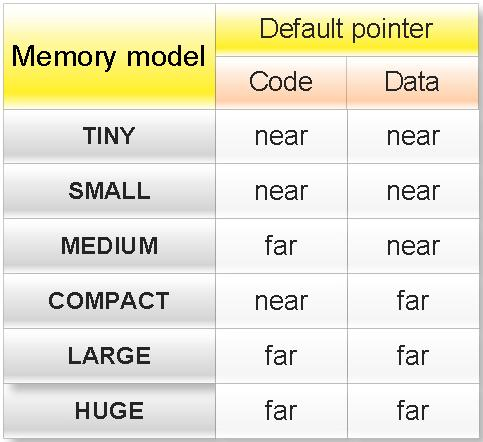## INDEX

### Memory model in c programming

Memory model:

In c there are six type of memory model.
If you want to see all memory model in Turbo C++ IDE then open Turbo C++ IDE and the go:
Options menu -> Compiler -> Code generation

These memory models are:

(a) TINY
(b) SMALL
(c) MEDIUM
(d) COMPACT
(e) LARGE
(f) HUGE

If you want to change the memory model then go to:

Options menu -> Compiler -> Code generation

And select any memory model and click OK button.Properties of memory mode in C:

(1) Memory model decides the default type of pointer in C.

Note:

Code: A pointer to function is called code.
Data: A pointer to variable is called data.

Examples:

(1)What will be output of following c program?

#include<stdio.h>
int main(){

int *ptr;
printf("%d",sizeof ptr);

return 0;
}

Output: Depends upon memory model.
Explanation: If memory model is TINY, SMALL or MEDIUM then default pointer will near and output will be 2 other wise output will be 4.

(2)What will be output of following c program?

#include<stdio.h>
int main(){

char (*fun)();
printf("%d",sizeof fun);

return 0;
}

Output: Depends upon memory model.
Explanation: fun is pointer to function. If memory model is TINY, SMALL or COMPACT then default pointer will near and output will be 2 other wise output will be 4.

(3)What will be output of following c program?

#include<stdio.h>
int main(){
int near *p,*q;
printf("%d , %d",sizeof(p),sizeof(q));

return 0;
}

Output: 2, Depend upon memory model.
Explanation: p is near pointer while type of pointer q will depend what is default type of pointer.

(4)What will be output of following c program?

#include<stdio.h>
int main(){
char huge **p;
printf("%d , %d",sizeof(p),sizeof(*p));

return 0;
}

Output: 4, Depend upon memory model.
Explanation: p is huge pointer while type of pointer *p will depend what is default type of pointer.

(5)Write a c program to find the memory model of you computer?

#include<stdio.h>
int main(){
#if defined __TINY__
printf("Memory model is: TINY");
#elif defined __SMALL__
printf("Memory model is:SMALL ");
#elif defined __MEDIUM__
printf("Memory model is:MEDIUM ");
#elif defined __COMPACT__
printf("Memory model is:COMPACT ");
#elif defined __LARGE__
printf("Memory model is:LARGE ");
#elif defined __HUGE__
printf("Memory model is:HUGE ");
#endif

return 0;

}

(2) Memory models decide the default size of segment.

Generic pointer
Null pointer
Wild pointer
Dangling pointer
Near pointer
Far pointer
Graphics video memory
Text video memory
Huge pointer
Memory model in C
C tutorial